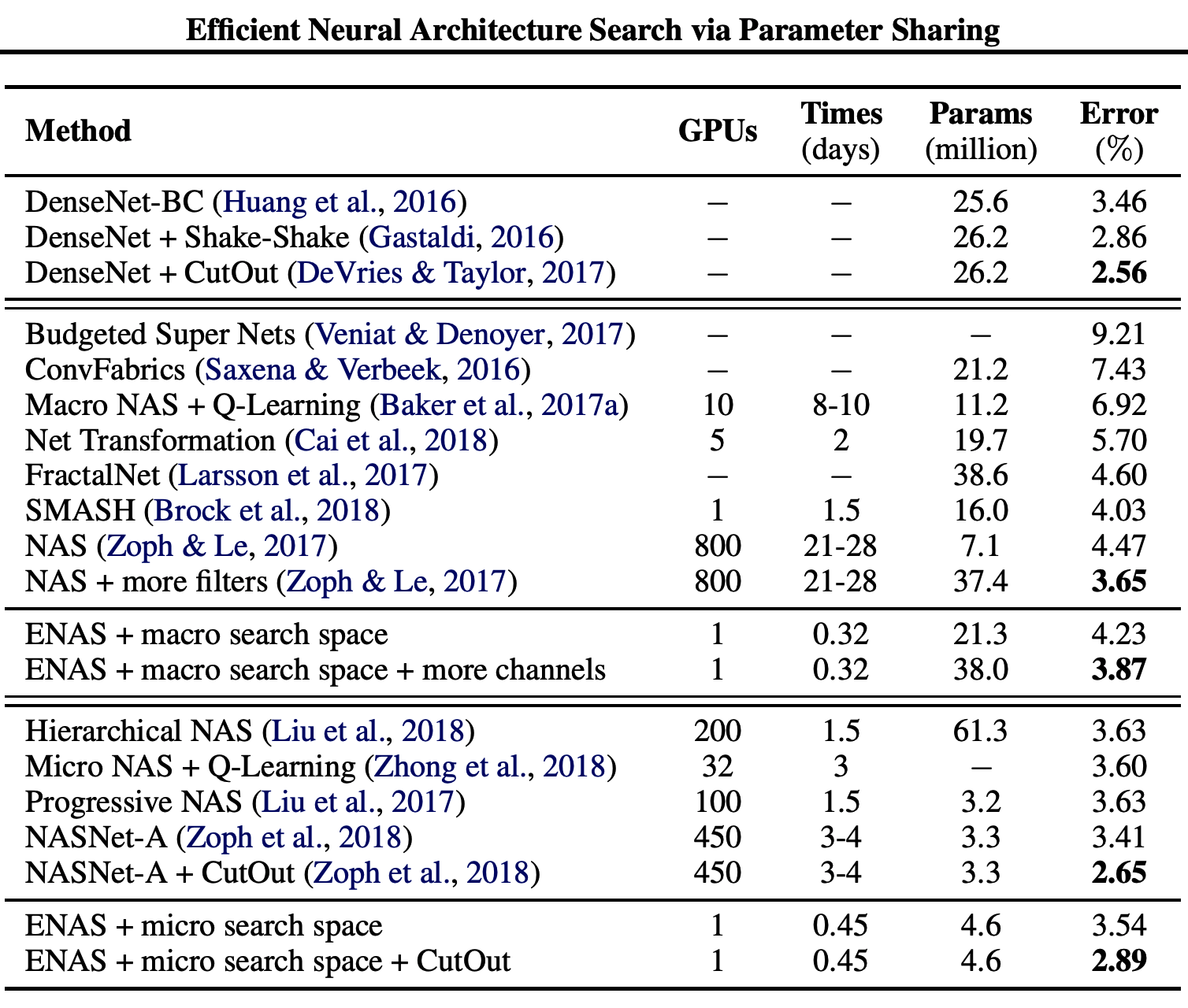# NAS

Author:张一极

2020-0127-1:55

## 主要结构和强化学习优化算法的推导

NAS目前主要分为了三个部分的内容

1. 搜索空间

首先是网络在搜索空间里采样出一个子网络结构，而后交给性能评估函数进行评估，返回结果后按照网络性能进行梯度估计和参数更新

重新采样网络，Step by Step的一个过程

搜索空间包括但不限于：DilConv，conv，pooling，bn，SepConvNxN（多DilConv），Fc，skip-connect等op的一些操作，定义了网络的基本算子

2. 搜索策略

随机（random）

RL（主流）

3. 评估指标

精度期望（单次精度波动存在且不确定）

# RL的梯度计算：

RNN作为控制器，每一次循环输出一个网络结构及其参数（多网络，主流方法是一个网络采样一种基本结构，两种网络采样两个结构作为基本的block），然后不同堆叠，组成子网络。

细节：因为每一个子网络在数据集上的表现波动很大，而进行多次实验的资源很浪费，故而使用子网络在数据集上的精度期望作为评价指标，（大数定律表明多次实验的结果依照概率收敛于期望），我们使用对于精度的无偏估计作为指标，$L(\theta)=E_{\delta \sim p_{\theta(\delta)}}[R(\delta)]$$E_{\Delta \sim p_{\theta(\delta)}}R（\Delta）$表示子网络在验证数据集上的精度期望，L的直观感受就是，精度期望越高，生成概率越高。

得到$L(\theta)=E_{\delta \sim p_{\theta(\delta)}}[R(\delta)]$，代表$\delta$为参数的p分布下，生成的$\delta$网络在数据集上的精度期望，这个期望越高，代表其效果越好，另一种表达方式是，一个序列动作中，即一连串的网络结构中，每一个结构获得的reward最终加和得到最后的期望。

梯度计算过程：

我们定义好整个序列动作和reward为$\tau=\left\{s_{1}, a_{1}, r_{1}, s_{2}, a_{2}, r_{2}, \cdots, s_{T}, a_{T}, r_{T}\right\} R(\tau)=\sum_{n=1}^{N} r_{n}$，这部分的R代表了整个序列动作得到的所有reward，但是有时候的情况是，动作序列和得到的reward有很多很多种，无法穷举计算，这时候我们一般采用大数定律做一个概率性的收敛得到一个估计值：$\bar{R}_{\theta}=\sum_{\tau} R(\tau) P(\tau \mid \theta)$，这个估计值代表了在$\tau$次的实验下，每一次实验得到的reward和出现这个结果的概率，这是可以穷举的，所以我们在这里做了一个近似。

我们的目的，就是最大化这个$\bar{R}_{\theta}=\sum_{\tau} R(\tau) P(\tau \mid \theta)$，我们就需要知道他的梯度。

首先，这个函数对参数的一阶导的话，第一项是没有意义的，因为和参数无关，只和动作结果有关，只需要对第二项微分即可：$\frac{\bar{R}_{\theta}=\sum_{\tau} R(\tau) P(\tau \mid \theta)\nabla \bar{R}_{\theta}}{d\theta}=\sum_{\tau} R(\tau) \nabla P(\tau \mid \theta)$，接着我们对这个函数作如下变形，为了得到一个关于P的式子，$\nabla \bar{R}_{\theta}=\sum_{\tau} R(\tau) \nabla P(\tau \mid \theta)=\sum_{\tau} R(\tau) P(\tau \mid \theta) \frac{\nabla P(\tau \mid \theta)}{P(\tau \mid \theta)}$，利用$\frac{\operatorname{d(log}(f(x))}{d x}=\frac{f'(x)}{f(x)}$，得到$\sum_{\tau} R(\tau) P(\tau \mid \theta) \nabla \log P(\tau \mid \theta)$，前半部分$\sum_{\tau} R(\tau) P(\tau \mid \theta)$，可以使用概率似然估计为$\sum_{\tau} R(\tau) P(\tau \mid \theta) \nabla \log P(\tau \mid \theta)\approx \frac{1}{N} \sum_{n=1}^{N} R\left(\tau^{n}\right) \nabla \log P\left(\tau^{n} \mid \theta\right)$

$\tau=\left\{s_{1}, a_{1}, r_{1}, s_{2}, a_{2}, r_{2}, \cdots, s_{T}, a_{T}, r_{T}\right\}$

取对数得到：

其中$log(p\left(reward_{t}, model_{t+1} \mid model_{t}, a_{t}\right))$与数据集的难易程度有关，与参数其实无关，顾仅有的一部分为$\sum_{t=1}^{T}log(p(a_{t} \mid s_{t}))$，由此可得：

为了解决REINFORCE算法计算出的梯度值偏差问题，在计算梯度时减掉了均值b，最终得到的梯度函数为：

如果用$L(\theta)$来表述的话，即：$\frac{1}{m} \sum_{k=1}^{m} \sum_{t=1}^{N} \nabla_{\theta} \log p\left(a_{t} \mid a_{t-1: 1} ; \theta\right)\left(R_{k}-b\right)$，m为小batch的个数，N是线性串型网络的层数，此方法只能用于生成无跳层连接和concat的网络结构，计算量较大，有两种可以解决的方案，一种解决方案是对搜索空间进行裁切，固定某几种网络结构作为基本类型，堆叠成重复的网络结构，可以得到很多经典网络（Resnet，inception...）（来自Barret Zoph and Quoc V Le. Neural architecture search with reinforcement learning. ICLR 2016.）

以下是NASnet和ENAS的一些结构和训练细节。

# NASnet

## 结构

NASnet采取了这样的解决方案，推理出基本模块，进行堆叠，在小数据集上训练（mini-cifar），最后tranfer到imagenet上，Controler预测的是基本两种网络block，分别称为Normal Cell和Reduction Cell，适应不同特征层尺寸需要，因为我们的图像在数据流中需要做一些变化去获取不同尺寸的特征，normal cell不改变图像尺寸，reduction cell将图像尺寸减半，适应于tranfer learning的需要（不同scale的img输入流），

NASnet在原来的基础上，做了一个新的事情，就是更改了搜索空间和堆叠策略，改为搜索两个基本结构，普通的cell没有改变特征图大小的功能，Reduction Cell会改比那图像尺寸，通过一个序列神经网络，分别预测两种结构（一种网络预测两种结构），每一个神经元的输出做不同的事情第一个为网络选择一个隐含状态作为第1个输入，第二个选择一个隐含状态作为第二个输入，第三个神经元选择为第一个隐含状态（前面层的输出结果）做运算（激活，池化，卷积），第四个同理激活第二个隐含层的输出，最后为两个计算结果选择合并方式，合并得到一个输出。

如下：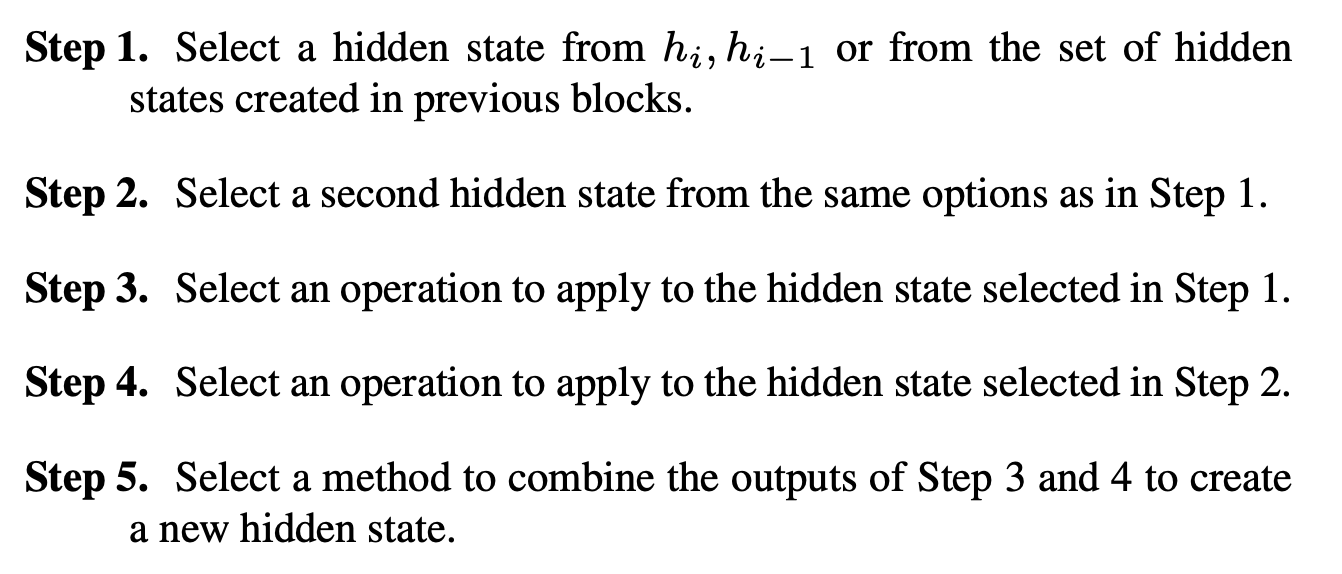(1) 选择一个隐含状态作为第1个输入

(2) 选择一个隐含状态作为第2个输入。 (3) 为第1个隐含状态选择一个运算。 (4) 为第2个隐含状态选择一个运算。 (5) 为两个运算的结果选择一个合并方式，执行合并。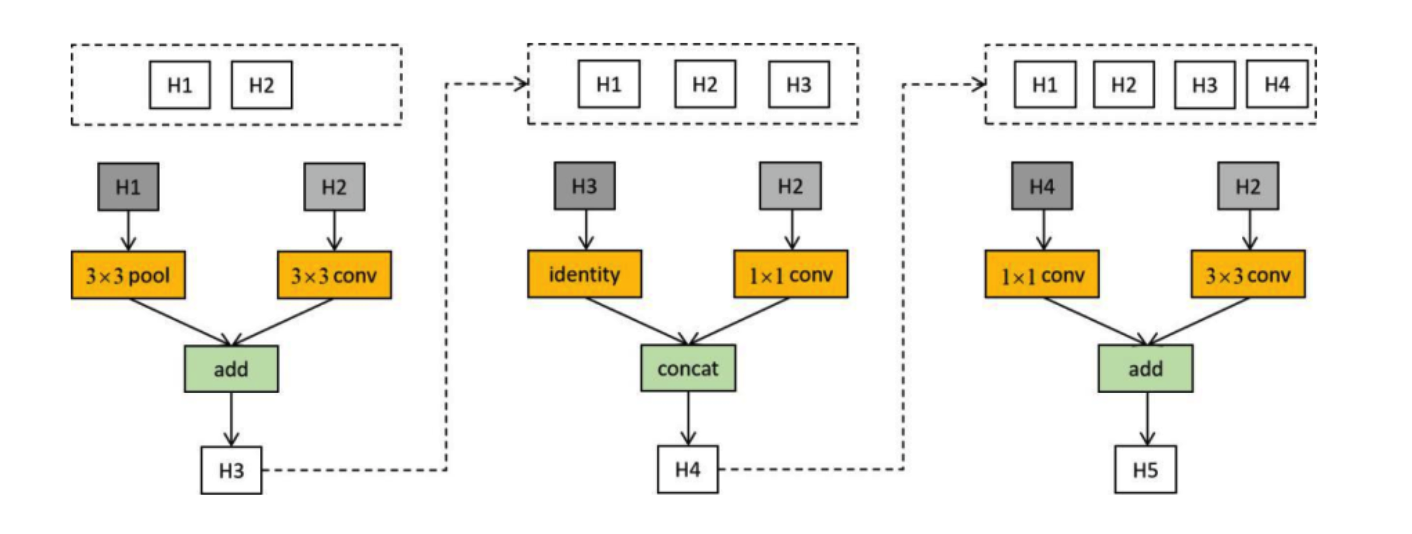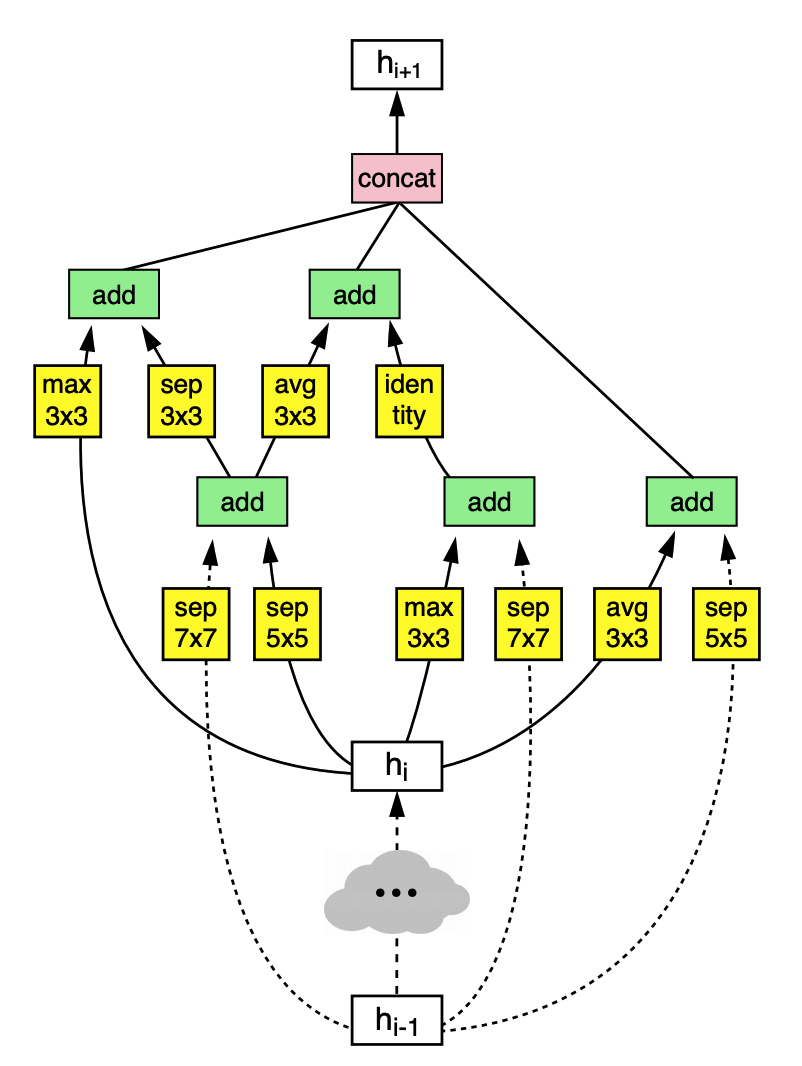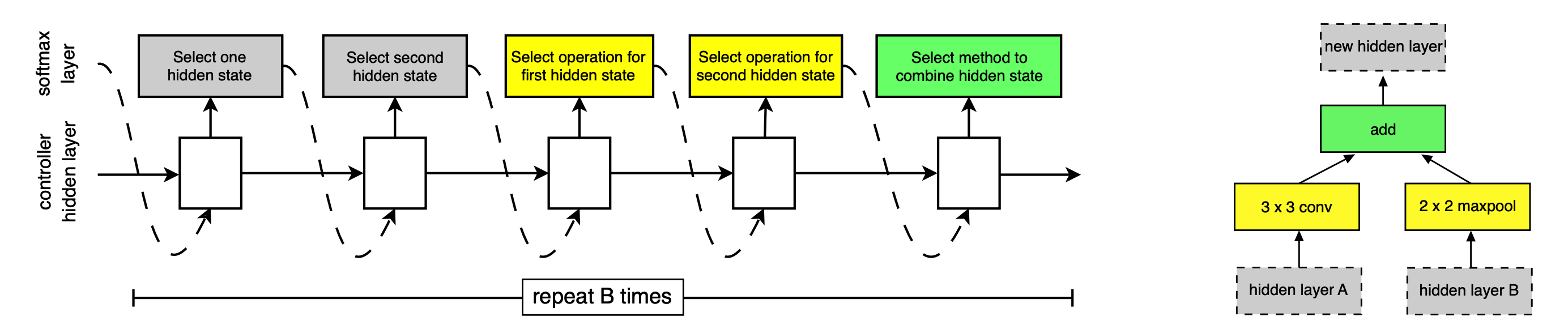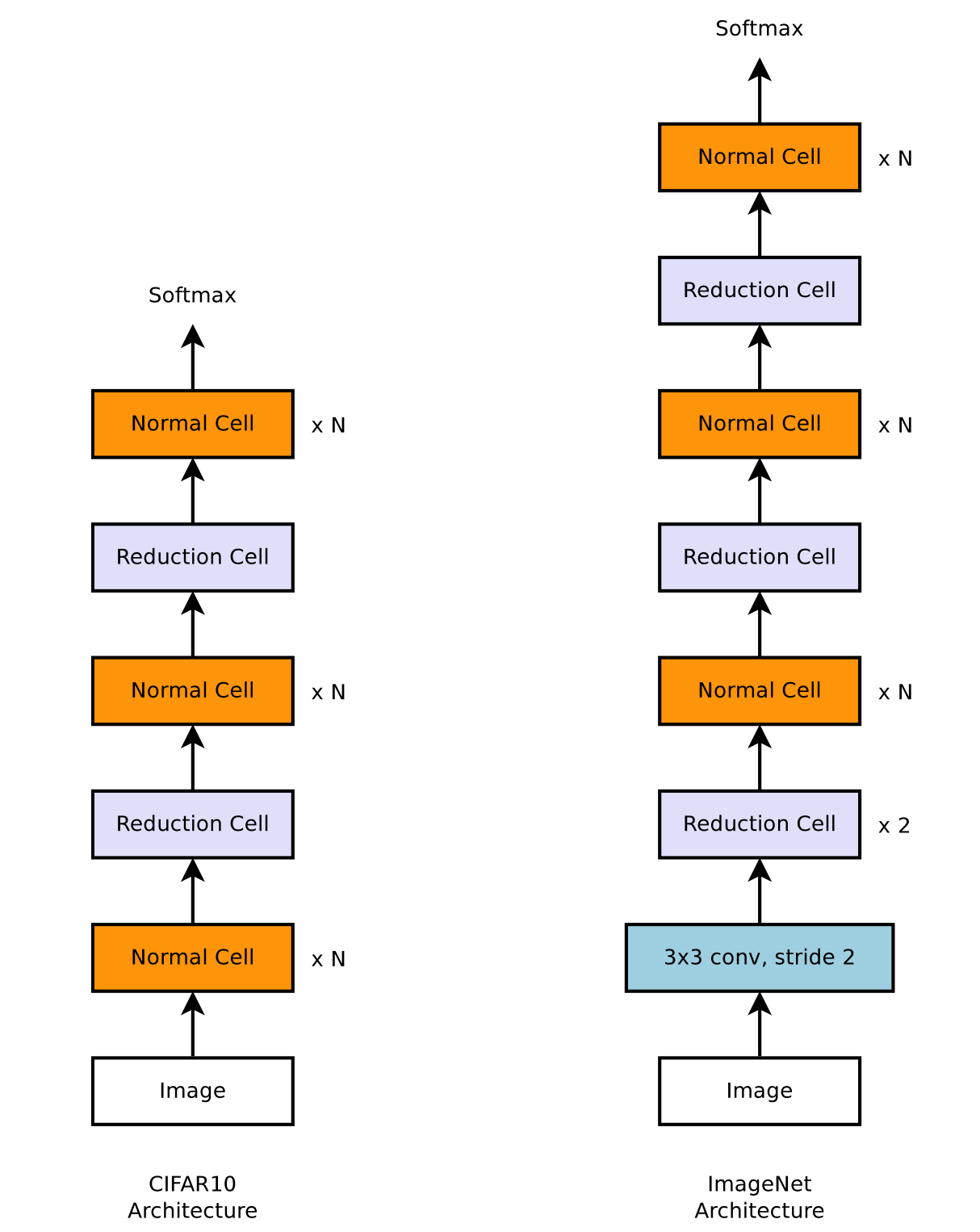## 效果：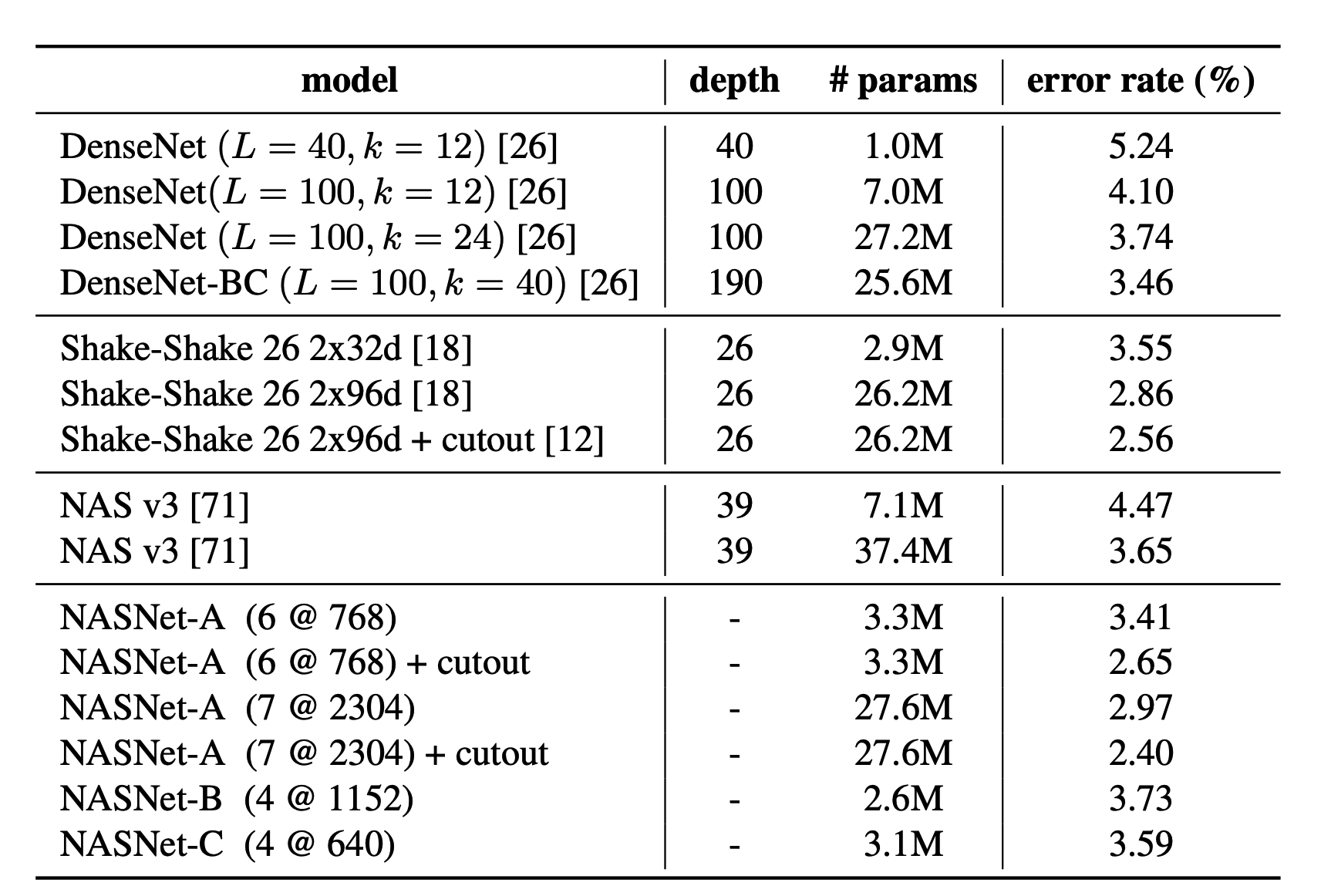### 准确率和参数量曲线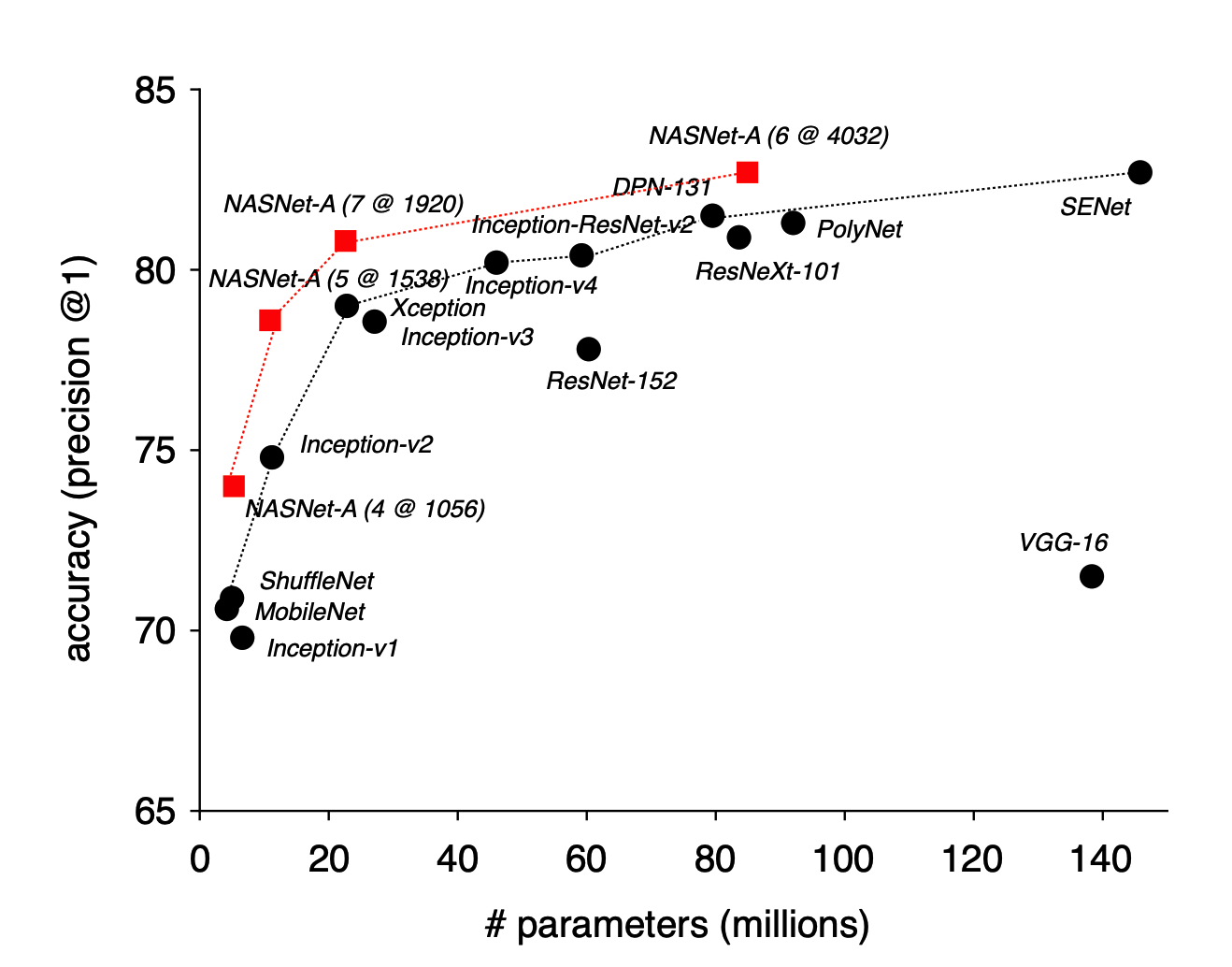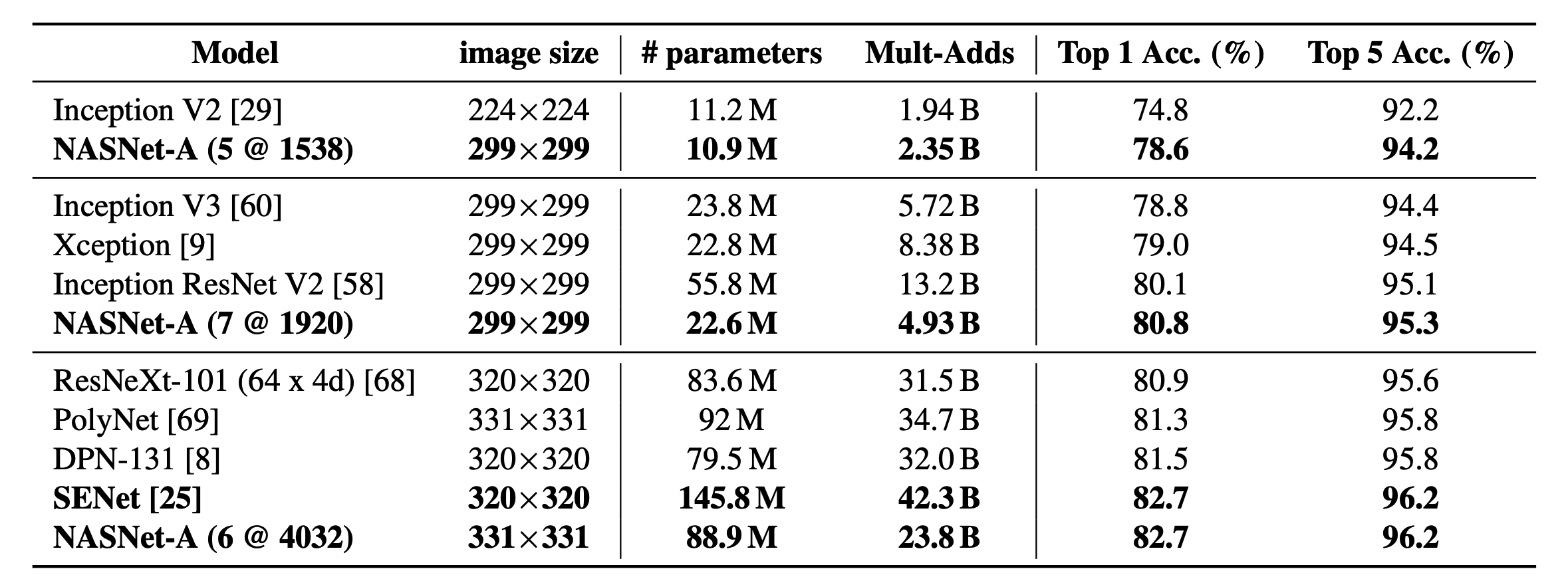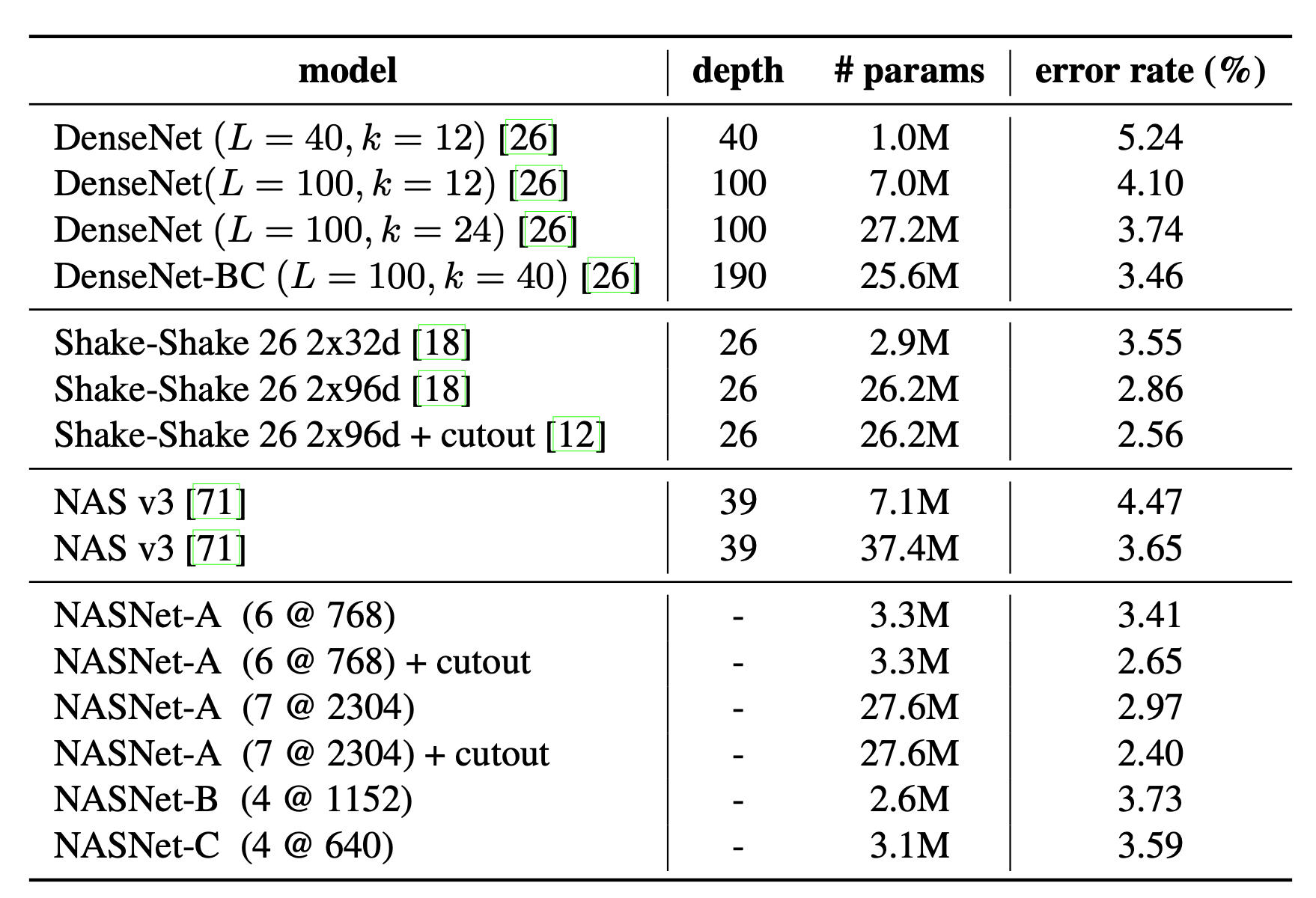NASNet-A(7@2304) 模型将错误率降低至 2.4%，超越了包括 DenseNet 和 Shake-Shake 在内的现有模型。其中，7代表 N=7，2304代表卷积神经网倒数第二层的过滤器数量为2304。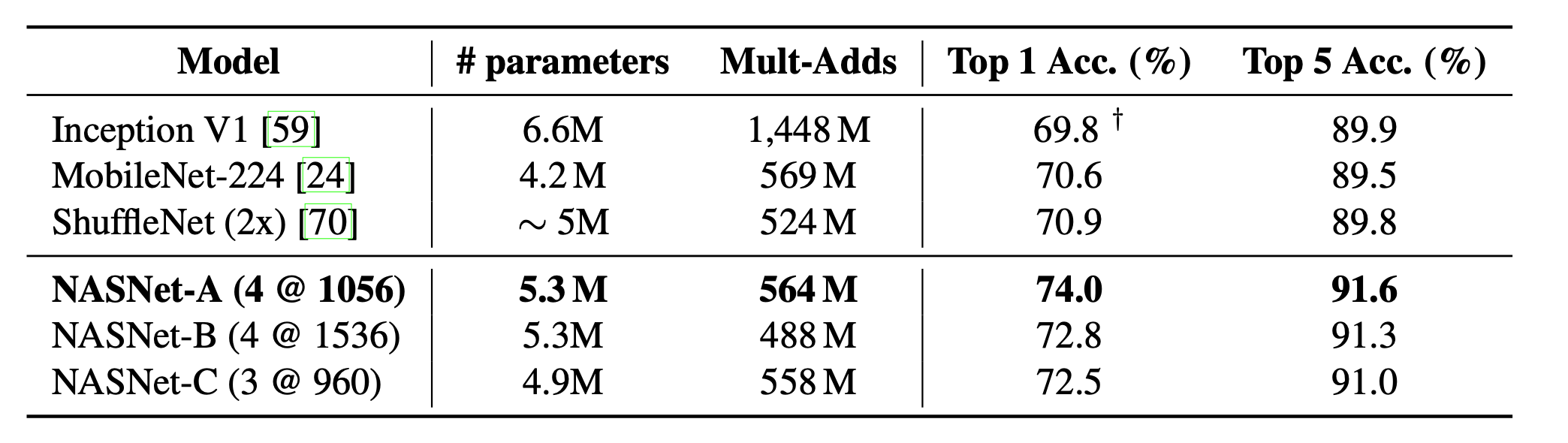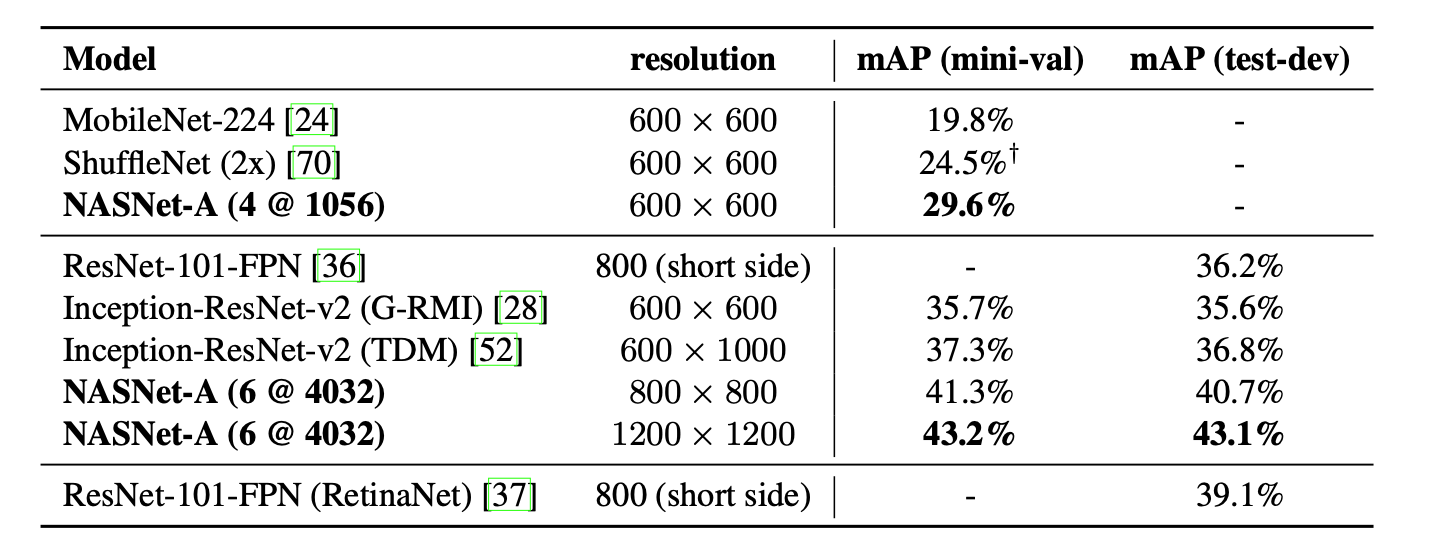# ENAS

## 定义搜索空间

ENAS的思路是将目前神经网络的搜索架构表现为有向无环图，即输入为有边射入，输出为有边射出，节点为处理算子的形式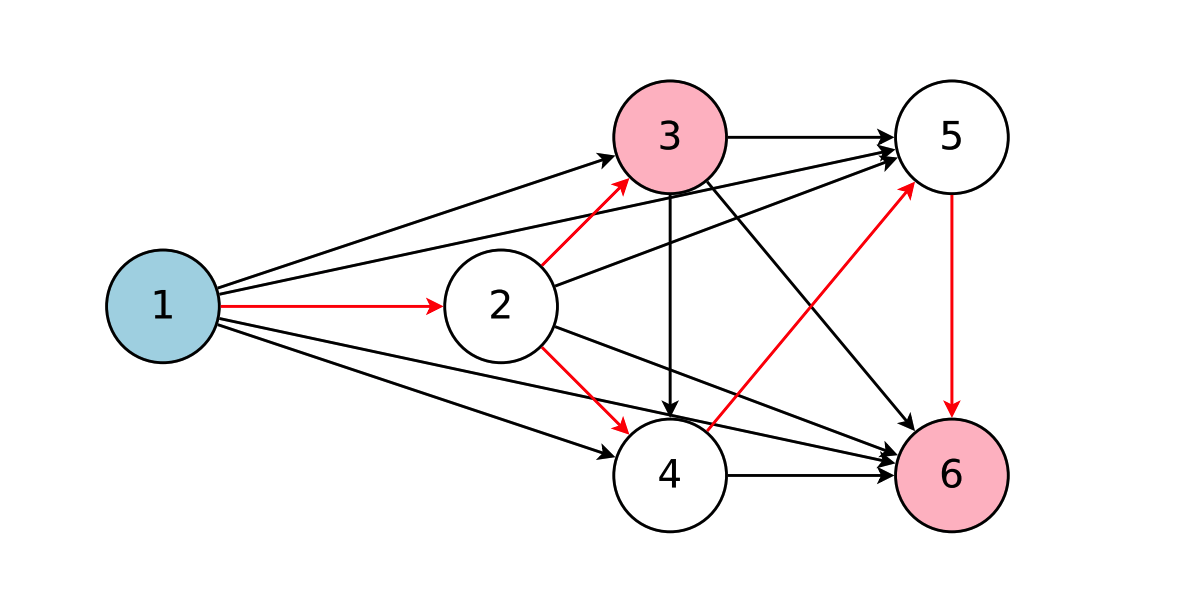ENAS使用一个RNN（称为controller）决定每个节点的计算类型和选择激活哪些边，ENAS中使用节点数为12的搜索空间，计算类型为tanh, relu, identity, sigmoid四种激活函 数，所以搜索空间有 $4^{N} \times N ! \quad$$10^{15}$ 种神经网络架构。

## 搜索过程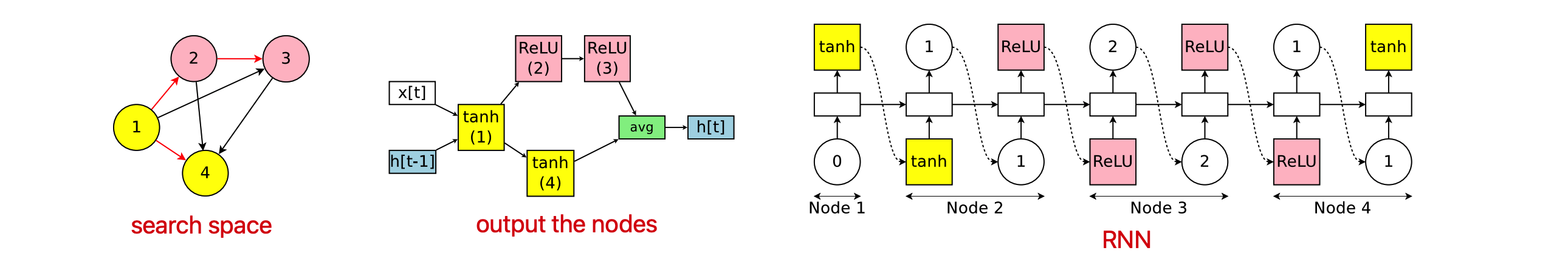### 设计卷积网络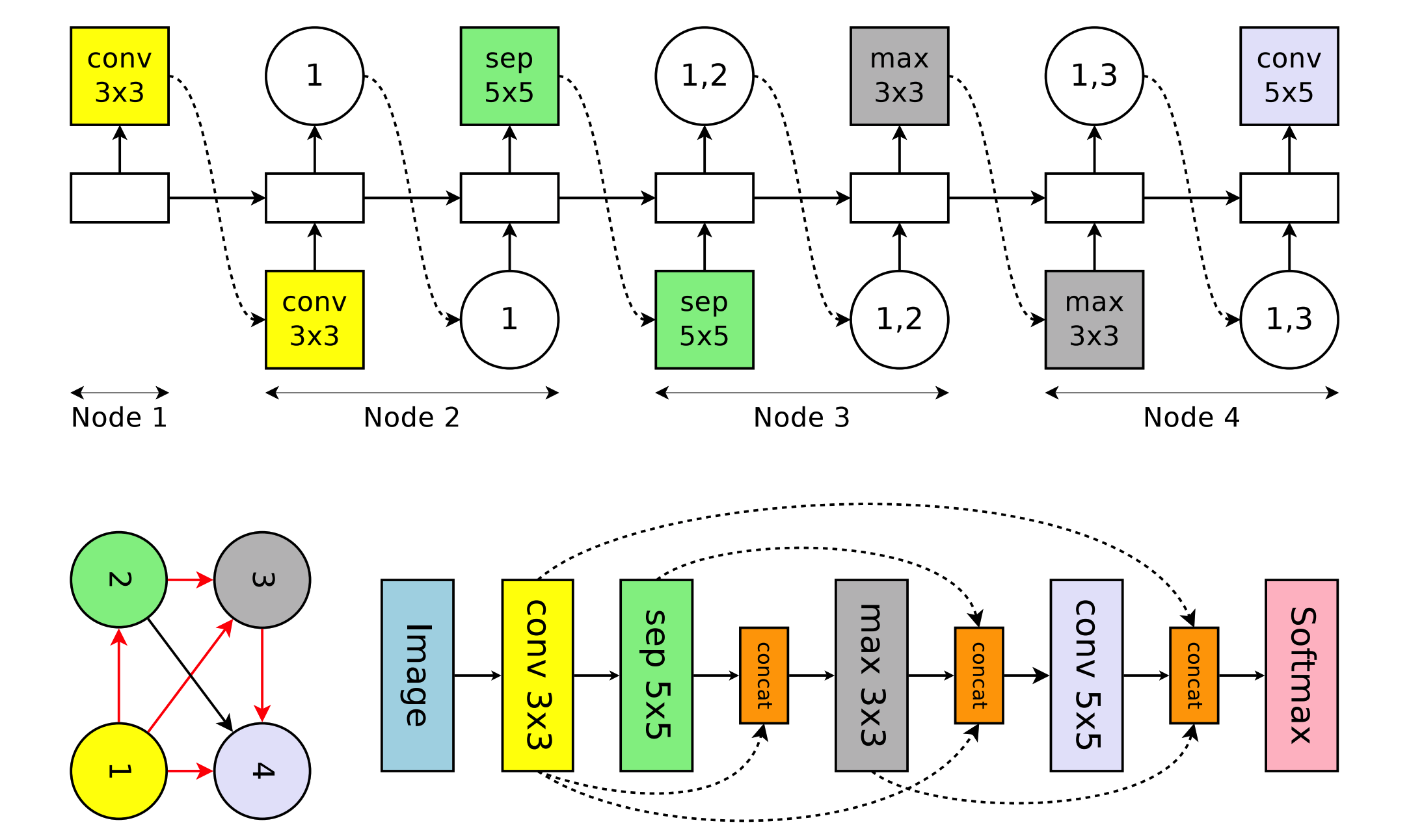### 设计卷积的block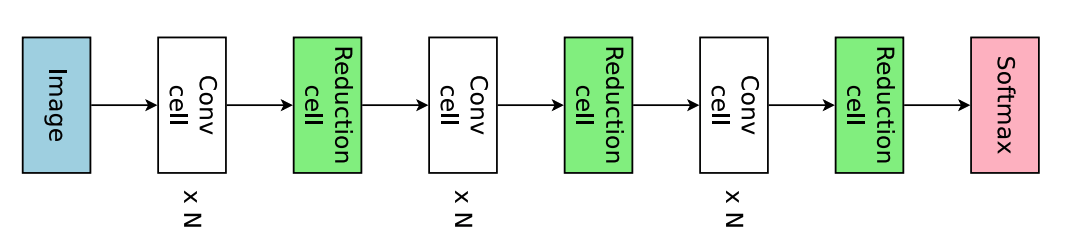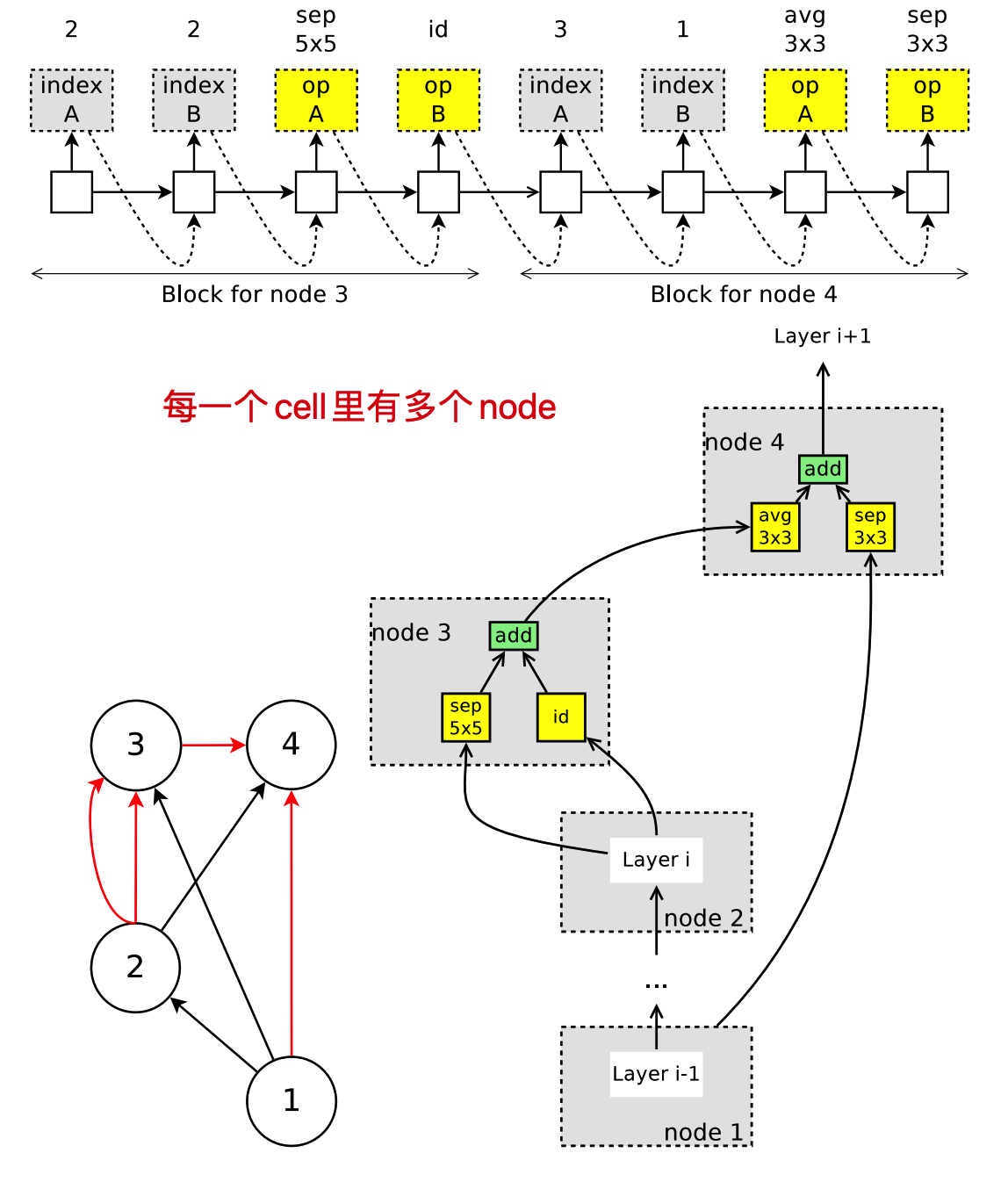ENAS 生成的所有模型节点数是一样的，而且节点的输入和输出都是一样的，所有模型的所有节点的所有权重都是可以加载来复用的，因此我们只需要训练一次模型得到权重后，让各个模型都去验证集做一个预估，只要效果好的说明发现了更好的模型了。实际上这个过程会进行很多次，而这组共享的权重也会在一段时间后更新，例如我找到一个更好的模型结构了，就可以用这个接口来训练更新权重，然后看有没有其他模型结构在使用这组权重后能在验证机有更好的表现。

## 训练细节

• 子网络模型的共享参数,用 $w$ 表示

• controller网络(即RNN（lstm）网络参数)

而训练ENAS的步骤主要包含两个交叉阶段：第一部训练子网络的共享参数 $w$;第二个阶段是训练controller的参数 $\theta$ 。这两 个阶段在ENAS的训练过程中交替进行，具体介绍如下：

1.共享参数的训练

2.训练controller参数θ

3.总体描述

## 结果

ENAS的结果没有NASnet好，这是因为ENAS没有像NAS那样从训练后的controller中采样多个模型架构，然后从中选出在验证集上表现最好的一个。